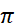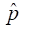Exam 1 Review Problems

1) Suppose that the observational units in a study are the patients arriving at an emergency room in a given day. For each of the following, indicate whether it can legitimately be considered a variable or not. If it is a variable, classify it as categorical (and if it is binary) or quantitative. If it is not a variable, explain why not.

a. Blood type

b. Waiting time

c. Mode of arrival (ambulance, personal car, on foot, other)

d. Whether or not men have to wait longer than women

e. Number of patients who arrive before noon

f. Whether or not the patient is insured

g. Number of stitches required

h. Whether or not stitches are required

i. Which patients require stitches

j. Number of patients who are insured

k. Assigned room number

2) When a tennis racquet is spun, is it equally likely to land with its label facing up or down? (This technique is often used to decide who should serve first.) Or does the spinning process favor one outcome more than the other? A statistics professor once investigated this question by spinning his tennis racquet many times. For each spin he recorded whether the racquet landed with the label up or down.

(a) Describe (in words) the relevant parameter whose value is being investigated with this study.

(b) Write the appropriate null and alternative hypotheses (in symbols).

He spun his racquet 100 times, finding that it landed with the label up in 46 of those spins.

(c) Would you consider these 100 spins to be a sample from a random process or a random sample from a population? Explain briefly.

(d) Describe how you could use a coin to conduct a simulation analysis of whether this result constitutes strong evidence that his racquet spinning process is not equally likely to land with its label facing up or down. Provide enough detail that someone else could implement the simulation and draw the appropriate conclusion.

(e) Use technology to simulate 1,000 repetitions of 100 spins each. Use the simulation result to produce an approximate p-value. Be very clear how you are carrying out this simulation and how you are finding the approximate p-value.

(f) Use the binomial distribution to calculate the p-value exactly. (Be sure to indicate how you calculate this probability: what values you use for n and, and what region you find the probability of.)

(g) Check whether the normal approximation (Central Limit Theorem) is valid here.

(h) Describe what the CLT says about the (approximate) sampling distribution of the sample proportion, assuming that the null hypothesis is true. Be sure to describe each of shape, mean, and standard deviation, and to include a rough sketch (but well labeled) of the distribution.

(i) Calculate and interpret the test statistic by finding the z-score for the observed sample proportion.

(j) Determine the (approximate) p-value from the standard normal distribution.

(k) What test decision would you make at the .05 significance level?

(l) Do the conditions for the (Wald) normal-based confidence interval hold here?

(m) Produce and interpret a 95% confidence interval for the parameter, using the Wald procedure if the conditions are met but using the Adjusted Wald procedure if they are not met.

(n) Is the confidence interval consistent with the test decision? Explain.

(o) Summarize your conclusion about the original question that motivated this study (be sure to comment on significance, confidence, and generalizability).

(p) Summarize how your calculations and conclusions would change if you instead examined the 54 spins that landed label down.

(q) Use the binomial distribution to determine the rejection region (in terms of number of “up” results in the sample) for the .05 significance level.

(r) Use the binomial distribution to determine the power of this test, using the .05 significance level, when the actual probability of this spun tennis racquet landing “up¨ is .65.

(s) Use the normal approximation to determine the rejection region (in terms of the sample proportion) for the .05 significance level.

(t) Use the normal approximation to determine the power of this test, using the .05 significance level, when the actual probability of this spun tennis racquet landing “up¨ is .65.

(u) How would the power in (t) change if you changed the level of significance to .01? You should explain/justify without performing any additional calculations.

(v) Use the normal approximation to determine how large the sample size n needs to be in order for the 95% confidence interval to have margin-of-error < .08.

3) Findings at James Madison University indicate that 21% of students eat breakfast 6 or 7 times a week.  A similar question was asked of a random sample of 159 Cal Poly students. Of the 97 who responded, 35 reported eating breakfast 6 or 7 times a week.  Is this convincing evidence that Cal Poly students have healthier breakfast habits (i.e., more likely to eat breakfast) than James Madison students?  More specifically, are you convinced that more than 21% of all Cal Poly students eat breakfast 6 or 7 times weekly?

(a) Define the population of interest and the sample being considered.

(b) Define the parameter and the statistic for this study.

(c) Estimate a p-value for addressing this question. Clearly explain how you are doing so and report this p-value.

(d) What conclusion would you draw from this p-value?

(e) Provide an interpretation of this p-value as if to someone not taking a statistics class.

(f) If you took another random sample of 159 Cal Poly students, which of your answers to part b would change?

(g) What are your thoughts about the fact that only 97 out of the original random sample of 159 responded?

(h) Suppose you plan to conduct a new study with a simple random sample of 1,590 Cal Poly students. Explain how you could obtain this sample.

(i) Would this new sample size address the issue you identified in part g?

(j) How would you expect this p-value in part c to change if of the 1,590 Cal Poly students you sample 36% reported eating breakfast 6 or 7 times a week (larger, smaller, or about the same)? Explain (without finding a new p-value!).

4) A plastics manufacturer will change the warranty on his plastic trash cans if the data from 20 cans strongly suggests that fewer than 90% of such cans would survive the 6-year warranty period.

(a) How many cans would need to survive to convince you to reject the null hypothesis?

(b) What is the power against the alternative value of 80%?